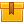# Introduction to Control Charts (A8502)

## Prerequisites

This lesson is designed for participants familiar with the basics of statistical process control. A knowledge of basic mathematical skills is recommended.

## Description

This lesson introduces control charts and shows how to plot specific values on the control chart. The lesson also demonstrates how to determine and plot the mean, median, and range on a control chart.

## Objectives

Explain the use of common elements of a control chart. Explain the two ways in which a process curve can change and describe the measures that are used to monitor those changes. Calculate and plot the mean using values with decimal points, both positive and negative numbers, and a scale marked either with actual values or increments above and below zero. Determine and plot the median. Calculate and plot the range using values with decimal points, and positive and negative numbers. Explain the difference between common and special causes of variations. Prerequisites: This lesson is designed for participants familiar with the principles of statistical process control, the basic components of control charts, and the characteristics of a normal curve. Knowledge of basic mathematical skills is recommended.

## ContentThis lesson introduces control charts and shows how to plot specific
values on the control chart. The lesson also demonstrates how to
determine and plot the mean, median, and range on a control chart.

•Introduction to Control Charts

0
0 Reviews